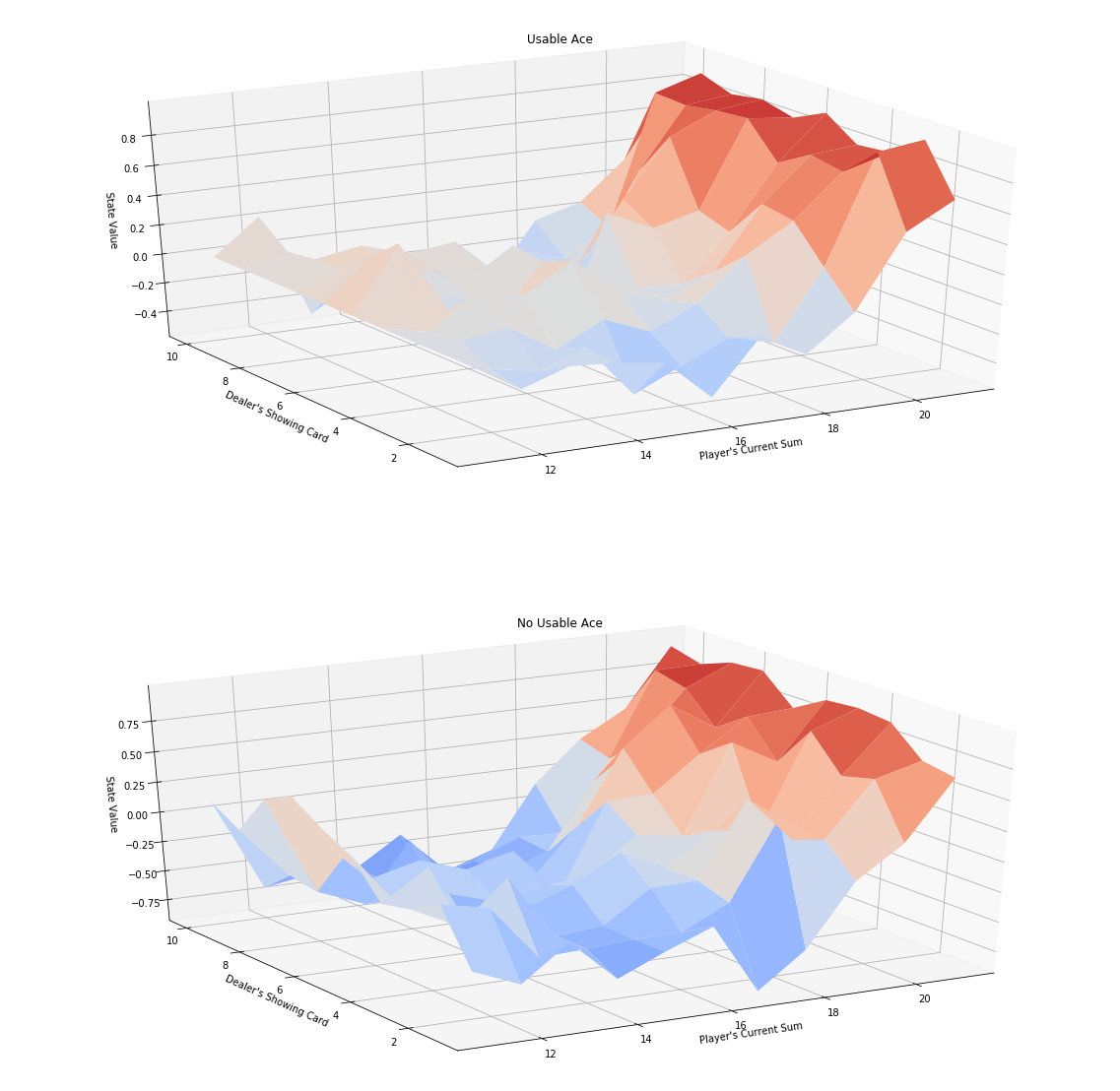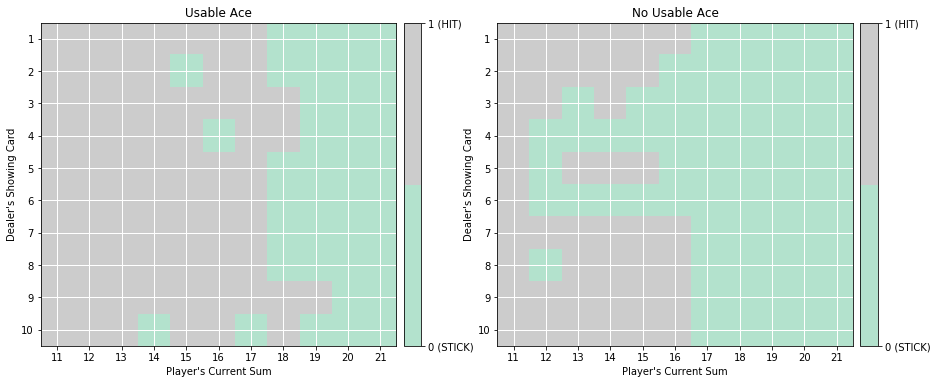# Monte Carlo Methods¶

In :
import sys
import gym
import numpy as np
from collections import defaultdict

from plot_utils import plot_blackjack_values, plot_policy

In :
env = gym.make('Blackjack-v0')


### MC Control¶

The algorithm implemented is the Every-visit Monte Carlo Method

The algorithm has four arguments this time:

• env: Instance of an OpenAI Gym environment.
• num_episodes: Number of episodes that are generated through agent-environment interaction.
• alpha: Step-size parameter for the update step.
• gamma: Discount rate for the rewards.

The algorithm returns as output:

• Q: Python dictionary (of one-dimensional arrays for the actions) where Q[s][a] is the estimated action value corresponding to state s and action a.
• policy: Pythin dictionary where policy[s] returns the action that the agent chooses after observing state s.

The pseudocode from the Sutton and Barto book for the following implementaiton is:In :
def mc_control(env, num_episodes, alpha, gamma=0.9, eps0=1.0, eps_decay=.99999, eps_min=0.05):

# Variable to store all the possible actions of the environment
nA = env.action_space.n

# Initialize empty dictionary of arrays
Q = defaultdict(lambda: np.zeros(nA))

# Initialize epsilon
eps = eps0

# Loop over episodes
for i_episode in range(1, num_episodes+1):

if i_episode % 1000 == 0:
print("\rEpisode {}/{}.".format(i_episode, num_episodes), end="")
sys.stdout.flush()

# Recalculate epsilon with a scheduler (a simple decay)
eps = max(eps*eps_decay, eps_min)

# Run the episode by following the eps-greedy policy
episode = generate_episode_from_Q(env, Q, eps, nA)

# Update the Q-Table values
Q = update_Q(env, episode, Q, alpha, gamma)

# Unroll our Q-Table picking the best action at each state (row) to define the found optimal policy
policy = dict((k,np.argmax(v)) for k, v in Q.items())

return policy, Q


We need to implement the wrapper functions we have defined in the control method above:

In :
def generate_episode_from_Q(env, Q, eps, nA):
'''
Function to generate a MC episode given the environment, the last Q-Table,
the ratio of exploration and the total number of actions
Returns: and episode as a 3-tuple of (states, actions, rewards)
'''
# Initialize an empty env to run the new episode
episode = []
state = env.reset()

# Until terminal state
while True:

# Generate an action following the policy
action = np.random.choice(np.arange(nA), p=get_probs(Q[state], eps, nA)) if state in Q else env.action_space.sample()

# Perform the 3-tuple for that state - Every visit approach
next_state, reward, done, info = env.step(action)
episode.append((state, action, reward))

state = next_state
if done:
break

return episode


We need to define the function get_probs.
This functions simply picks one action among all the possible actions nA, based on the probabilities they have.
These probabilities are the values of the Q-Table for one particular row, corresponding to all the actions available for the state represented in that row.

In :
def get_probs(Qs, eps, nA):
'''
Function that obtains the probabilites corresponding to e-greedy policy
'''
# 1 - Initial equal radom probability for every possible action
policy_s = np.ones(nA) * eps / nA

# 2 - Determine which is the current optimal action for that state
best_a = np.argmax(Qs)

# 3 - Update (increase) the probability for the optimal action
policy_s[best_a] = 1 - eps + (eps / nA)

return policy_s


The implementation for the update rule:

In :
def update_Q(env, episode, Q, alpha, gamma):
'''
Function to update the Q-Table after running 1 episode
'''
# 1 - Extract the information of the run episode
states, actions, rewards = zip(*episode)

# 2 - Apply the discount factor
discounts = np.array([gamma**i for i in range(len(rewards)+1)])

# 3 - Apply the update function to every Q(s,a) <- Q(s,a) + alpha*[Gt - Q(s,a)]
for i, s in enumerate(states):

a = actions[i]
old_Q = Q[s][a]
Q[s][a] = old_Q + alpha*(sum(rewards[i:]*discounts[:-(1+i)]) - old_Q)
return Q

In :
# The value for alpha and the number of episodes have been set after trial and error
policy, Q = mc_control(env, 1000000, 0.1)

Episode 1000000/1000000.

Next, we plot the corresponding state-value function.

In :
# obtain the corresponding state-value function
V = dict((k,np.max(v)) for k, v in Q.items())

# plot the state-value function
plot_blackjack_values(V)Finally, we visualize the policy that is estimated to be optimal.

In :
# plot the policy
plot_policy(policy)The true optimal policy $\pi_*$ can be found in Figure 5.2 of the Sutton book (and appears below).